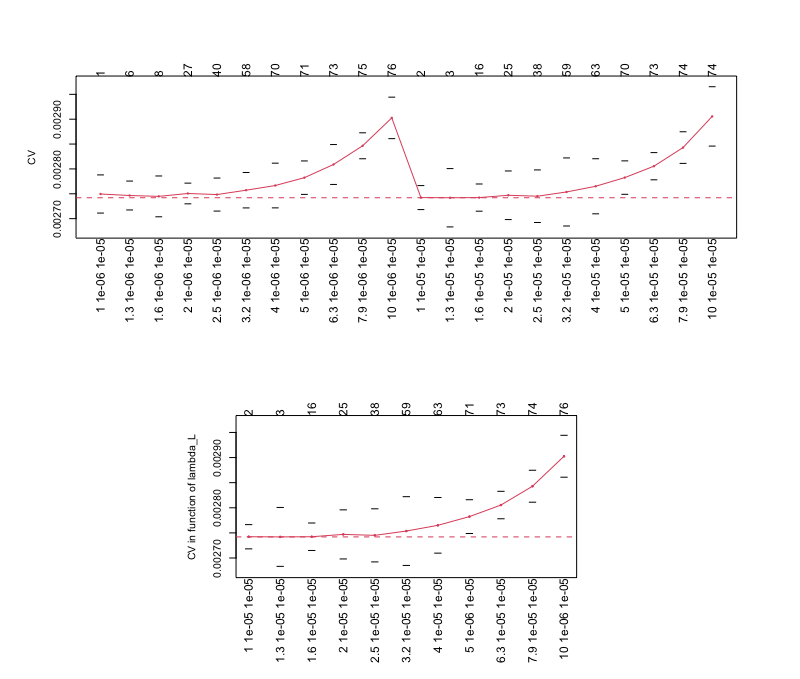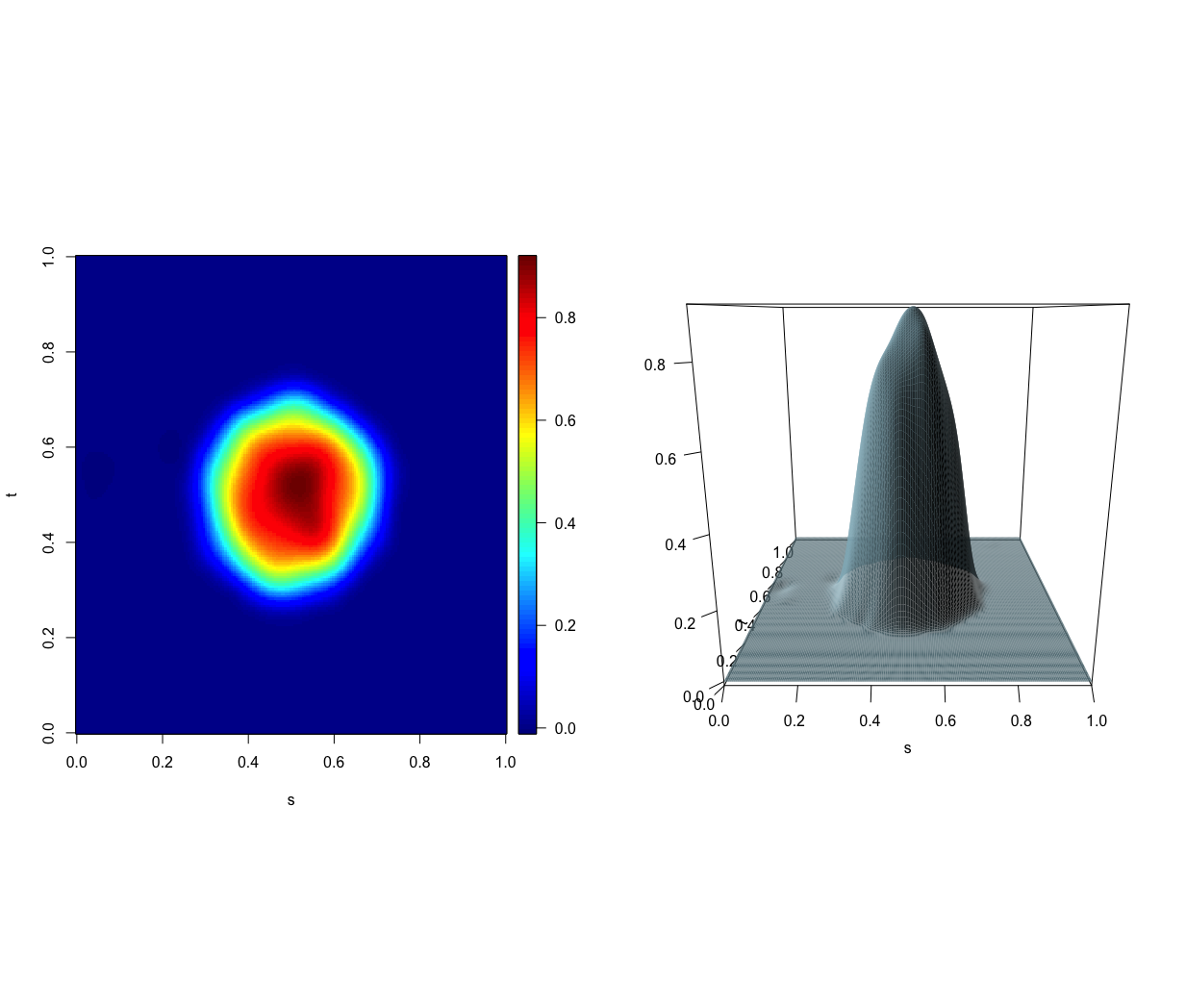# slasso

The package slasso implements the smooth LASSO estimator (S-LASSO) for the Function-on-Function linear regression model proposed by Centofanti et al. (2020). The S-LASSO estimator is able to increase the interpretability of the model, by better locating regions where the coefficient function is zero, and to smoothly estimate non-zero values of the coefficient function. The sparsity of the estimator is ensured by a functional LASSO penalty, which pointwise shrinks toward zero the coefficient function, while the smoothness is provided by two roughness penalties that penalize the curvature of the final estimator. The package comprises two main functions `slasso.fr` and `slasso.fr_cv`. The former implements the S-LASSO estimator for fixed tuning parameters of the smoothness penalties λs and λt, and tuning parameter of the functional LASSO penalty λL. The latter executes the K-fold cross-validation procedure described in Centofanti et al. (2020) to choose λL, λs, and λt.

## Installation

The development version can be installed from GitHub with:

``````# install.packages("devtools")
devtools::install_github("unina-sfere/slasso")``````

## Example

This is a basic example which shows you how to apply the two main functions `slasso.fr` and `slasso.fr_cv` on a synthetic dataset generated as described in the simulation study of Centofanti et al. (2020).

``library(slasso)``

Then, we generate the synthetic dataset and build the basis function sets as follows.

``````data<-simulate_data("Scenario II",n_obs=500)
X_fd=data\$X_fd
Y_fd=data\$Y_fd
domain=c(0,1)
n_basis_s<-30
n_basis_t<-30
breaks_s<-seq(0,1,length.out = (n_basis_s-2))
breaks_t<-seq(0,1,length.out = (n_basis_t-2))
basis_s <- fda::create.bspline.basis(domain,breaks=breaks_s)
basis_t <- fda::create.bspline.basis(domain,breaks=breaks_t)``````

To apply `slasso.fr_cv`, sequences of λL, λs, and λt should be defined.

``````lambda_L_vec=10^seq(0,1,by=0.1)
lambda_s_vec=10^seq(-6,-5)
lambda_t_vec=10^seq(-5,-5) ``````

And, then, `slasso.fr_cv` is executed.

``````mod_slasso_cv<-slasso.fr_cv(Y_fd = Y_fd,X_fd=X_fd,basis_s=basis_s,basis_t=basis_t,
lambda_L_vec = lambda_L_vec,lambda_s_vec = lambda_s_vec,lambda_t_vec =lambda_t_vec,
max_iterations=1000,K=10,invisible=1,ncores=12)``````

The results are plotted.

``plot(mod_slasso_cv)``By using the model selection method described in Centofanti et al. (2020), the optimal values of λL, λs, and λt, are 3.98, 10 − 5, and 10 − 5, respectively.

Finally, `sasfclust` is applied with λL, λs, and λt fixed to their optimal values.

``````mod_slasso<-slasso.fr(Y_fd = Y_fd,X_fd=X_fd,basis_s=basis_s,basis_t=basis_t,
lambda_L = mod_slasso_cv\$lambda_opt_vec,lambda_s = mod_slasso_cv\$lambda_opt_vec,
lambda_t =  mod_slasso_cv\$lambda_opt_vec,invisible=1,max_iterations=1000)``````

The resulting estimator is plotted as follows.

``plot(mod_slasso)``• Centofanti, F., Fontana, M., Lepore, A., & Vantini, S. (2020). Smooth LASSO Estimator for the Function-on-Function Linear Regression Model. arXiv preprint arXiv:2007.00529.